##Oil & Gas Apps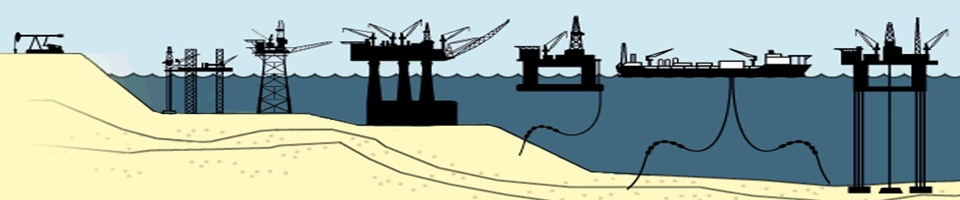Home  -  Gas-Leak - AGA-3  -  ISO-5167  - Restrict-Ori  -  Oil&GasPVT  - Privacy Policy

Easy to use engineering apps for the oil and gas industry - designed for practical field applications.
Apps have been tested and proven both on offshore and onshore oil and gas installations.

Designed for iPhone or iPad - available from the AppStore.

Available Apps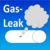Info

Gas-Leak calculates leak-rates from oil and gas leaks in oil and gas operations and equipment.

Gas-Leak - DocumentationFree iPhone App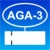Info

AGA-3 calculates size, flowrate or pressure drop for gas and liquid flow orifice meters based on the American Gas Association AGA report No. 3 (also API Manual of Petroleum Measurement Standards, Chapter 14.3).

AGA-3 - DocumentationAGA-3 on the App Store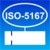Info
ISO-5167 calculates size, flowrate or pressure drop for gas and liquid flow orifice meters based on International Standard ISO-5167-2:2003.
ISO-5167 - DocumentationISO-5167 on the App Store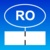Info
Restrict_Ori calculates size and flowrates for gas and liquid restriction orifice meters based on R.W. Miller's "Flow Measurement Handbook".
Restriction Orifice - DocumentationRestrict_Ori on the App Store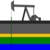Info
Oil&GasPVT calculates physical properties (PVT) of Gases (Natural Gas, Air and Nitrogen) and Liquids (Oil, Water, MeOH, MEG, DEG and TEG) based on published correlations.
Oil & Gas PVT - DocumentationFree iPhone App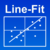Line-Fit performs straight line regression calculation for X, Y-value pairs by method of Least Squares.  Line-Fit - DocumentationFree iPhone App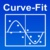Curve-Fit uses regression analysis by method of least squares to fit different equations to a data set.  Up to 10 different equations can be fitted to the data. Curve-Fit - DocumentationFree iPhone AppWe do not collect any usage data, personal information, or any other information from users.

Note that users must accept the Disclaimer/Warranty stated below.

This policy is effective as of 2021-05-21 and may be updated from time to time and any changes will be posted on this page.

Disclaimer/Warranty:

Users of the apps must accept this disclaimer of warranty:

Each app is provided "as is" without warranty of any kind, either expressed or implied, including any warranty of merchantability or fitness for a particular purpose.  In no event shall the Author/Developer be held liable for any loss of profit, special, incidental, consequential, or other similar claims.

Home

 Gas-Leak For natural gas the assumption is that the pressure difference across the leak-point (hole) is large enough to cause critical flow across the leak-point. Flowrate is calculated based on restriction orifice correlations, i.e., sonic velocity exists at the hole orifice and further decrease in the upstream and downstream pressures remain constant. Includes table of expected fugitive emission rates from equipment. For liquids, critical (choked) flow occurs when a cavitation barrier exists across the leak-point (hole) where downstream pressure (atmospheric) is below the liquid vapor pressure, at the point when the liquid will start to flash into bubbles of vapor. For crude oil with API gravity below about 35 and water, the vapor pressure will normally be less than atmospheric pressure.  Therefore, in this app, if vapor pressure is found to be lower than atmospheric pressure, the flowrate is calculated based on control valve pressure drop calculations  Gas Leak Calculations Gas leak rates are based on the assumption that the pressure difference across the leak-point (hole) is large enough to cause critical flow across the leak-point. Flow-rate is calculated based on restriction orifice correlations. This means that sonic velocity exists at the orifice throat, and further decrease in the downstream pressure does not increase mass flow rate. Flow-rate calculated is only correct if upstream and downstream pressures remain constant. In a blow-down situation the flowrate drops significantly due to reduction in upstream pressure until pressures equalize across the leak-point. In this case, the app only calculates the initial flowrate.  Restriction orifice calculations in this program are performed according to R. W. Miller's "Flow Measurement Engineering Handbook". For critical flow the basic mass flow rate equation is:                               𝑄𝑚 = 1336.485 x 𝐶 x 𝑑² x √𝑍 x 𝑌cr x √(𝜌 x FTP  x 𝑃) where                  Qm       =     mass flow rate, lbm /h                  C         =     critical discharge coefficient                  d          =     orifice diameter at flowing conditions, in                  YCR      =     critical flow function                   FTP        =     total pressure correction factor to adjust for difference between static pressure and total pressure of the fluid                   and      Z, r, and P are measured at flowing upstream conditions For ß-ratios less than 0.5 the total pressure correction factors are approximated by:                                            FTP = {1 - k/2[2/(k+1)][(k+1)/(k-1)] × ß4}-1                                                                                                   YCR = {k/Z × [2/(k+1)][(k+1)/(k-1)]}0.5                                                       where       k          =     isentropic coefficient at flowing conditions Assuming steady isentropic flow, critical flow (choked flow) occurs when:                                            P2/P1/FTP < [2/(k+1)][k/(k-1)]                                                                      where       P1            =     pressure upstream orifice                   P2            =     pressure downstream orifice By assuming sharp-edged orifices with plate thickness to bore diameter between 1 and 6 the discharge coefficient is a constant given in the program as:                                                                 C = 0.83932 The gas leak calculations also provide an overview of Fugitive Emission Factors for common equipment in the oil and gas industry, data from 2014, can be used as comparison with calculated leak-rates from the app.   Liquid Leak Calculations For liquids, choked flow occurs when a cavitation barrier exists across the hole where downstream pressure (atmospheric) is below the liquid vapor pressure.  At that point the liquid will partially flash into bubbles of vapor. This will normally be true for crude oil, but for water, the vapor pressure will be below atmospheric pressure.  Therefore, in this app, if vapor pressure is found to be lower than atmospheric pressure, the flowrate is calculated based on control valve pressure drop calculations as per Baumann.  The sizing and flowrate equation used for liquids is:                                                                Qm = C x E x ¶/4 x d² x √(2 x DP x 𝜌1)  where   DP       =          Pressure Drop, calculated as per above, depending on Pv and P2, Pa (Pascal) P1        =          Upstream orifice pressure, Pa P2        =          Downstream orifice pressure, Pa PV        =          Vapor Pressure, Pa                               𝜌1           =          density of fluid at upstream pressure, kg/m3                               E          =          velocity of approach factor = (1- ß4)-0.5 The discharge coefficient, C is determined empirically: C = 0.5961+0.0261ß2-0.216ß8+0.000521 × [106 x β/ReD]0.7+(0.0188+0.06A) x β3.5 × [106 /ReD]0.3         + (0.043+0.080e-10L1 - 0.216e-7L1) x (1-0.11A) x (β/(1-β4))-0.031(M’2-0.8M’21.1) x ß1.3 where             ReD          =          pipe Reynolds number             M’2         =          2L’2/(1-β)             A            =          [19000ß/ReD]0.8 Assuming square-edged orifices with plate thickness to bore diameter less than 6 with a minimum of 0.125 in (3 mm), the liquid restriction orifice constant used in this app is: C = 0.6             Home  -  Download
AGA-3 Orifice

AGA-3 Orifice calculations are based on the American Gas Association AGA report No. 3 (also API Manual of Petroleum Measurement Standards, Chapter 14.3). Orifice size is calculated from given flowrate and pressure drop, Flowrate is calculated from given size and pressure drop and Pressure Drop requires orifice size and flowrate. Different taps can be selected: Flange taps or Pipe taps. Fluids include gases: Natural Gas (Specific Gravity must be provided), Air and Nitrogen, or liquids: Crude Oil, Water, Methanol, Mono-ethylene Glycol, Diethylene Glycol and Triethylene Glycol. In addition, selecting orifice material will adjust the sizing parameter of the orifice depending on temperature.

Physical Properties
Standard conditions for AGA-3 Orifice are defined according to US Customary system as 60 °F (15.56 °C) and 14.73 Psia (1.0156 Bara). Customary units (US) (Temp = °F, Pressure = Psia) and SI-units (Temp = °C, Pressure = Bara) are supported.

For natural gas the AGA-8 equation-of-state (EOS) is used for calculating Z-factor (compressibility factor) specific heat capacity and isentropic coefficient. For air and nitrogen, the Redlich-Kwong EOS is used. Other physical properties are calculated from common empirical equations used in the oil and gas industry (viscosity, pseudo-critical properties).

For liquids, the physical properties are calculated from common empirical equations used in the oil industry.

Orifice Meters with Flange Taps:

The fundamental orifice meter flow equation is:

Q=359.072Cd(FT)EvY1d2𝜌1DP

where

Qm        = mass flow rate (lbm/hr)

359.072 = Conversion factor for flowrate in lbm/hr, d in inches, and DP in inches H2O

Cd(FT)  = coefficient of discharge for flange tapped orifice meters

Ev          = velocity of approach factor

Y1         = expansion factor (upstream tap)

d            = orifice plate bore diameter, inches

𝜌1          = density of fluid at upstream flowing conditions, lbm/ft3

DP         = orifice differential pressure, inches H2O at 60 °F

Cd(FT) = Ci(FT) + 0.000511 × (1,000,000 × ß/ReD)^0.7 + {0.0210 + 0.0049 × (19,000 × ß/ReD)^0.8} × ß4 × (1,000,000/ReD)^0.35

Ci(FT) = Ci(CT) + [(0.0433 + 0.0712 e -8.5L1 – 0.1145 e -6.0L1] × (1 – 0.23 × (19,000 × ß/ReD)^0.8) × β4/(1 – β4) - 0.0116 × {2/[D × (1-ß)] - 0.52 × (2/[D × (1-ß)])^1.3} × ß1.1 × {1 - 0.14 × (19,000 × ß/ReD)^0.8}

Ci(CT) = 0.5961+ 0.0291 × ß^2 - 0.2290 × ß^8

If pipe diameter is less than 2.8 inches, the Ci(CT) orifice calculation factor is modified as follows with D in inches:

Ci(CT) = Ci(CT) + 0.003 × (1-ß) × (2.8 - D)

ReD    = Reynolds number for the pipe, dimensionless = 𝜌1𝑣𝐷/μ

𝑣         = fluid velocity

D        = pipe diameter

μ         = viscosity

Ev       = velocity of approach factor = (1 - ß^4)^-0.5

Y1       = expansion factor (upstream tap) = 1 – (0.3625 + 0.1027×β^4 + 1.1320 × β^8) × {1 – [P2/P1]1/κ}

ß.         = diameter ratio, (d/D)

κ.         = isentropic coefficient

P1, P2 = pressure at upstream and downstream tap respectively

Orifice Meters with Pipe Taps:

Current AGA/API Standard does not contain details of pipe tap orifice metering, but refer to the 1992 Standard (3rd Edition), which uses the factor-method for calculation of flow orifices.

For gas orifices:

Q=C√(P1hw)

where

Qv = volumetric flowrate, ft3/hr
C* = orifice flow constant = Fb × Fr × Y1 × Fpb × Ftb × Ftf × Fgr × Fpv
P1 = upstream flowing pressure, psia
hw = orifice differential pressure, inches H2O at 60 oF
Y1 = expansion factor (upstream tap) = 1 - (0.333 +1.145×(β^2 + 0.7 × β^5 + 12 × β^12) × {(ΔP/P1 × 𝜅}

Fb   = basic orifice factor  = 338.178d2 K0
Fr    = Reynolds number factor = 1 + E/ReD

Fpb = base pressure factor
Ftb  = base temperature factor
Ftf   = flowing temperature factor
Fgr  = real gas relative density factor
Fpv  = super-compressibility factor
K0   = coefficient of discharge at infinite Reynolds number = Ke/[1 + 15 x E/1,000,000/d

Ke   = coefficient of discharge when Reynolds number = 1,000,000 x d/15
= 0.5925 + 0.0182/D + (0.44 - 0.06/D) × ß^2 + (0.935 + 0.225/D) × ß^5 + 1.35 × ß^14 + (1.43/D^0.5) × (0.25 - ß)^2.5

E     = d × (905 - 5000 × ß + 9000 × ß^2 - 4200 × ß^3 + 875/D)

ß     = diameter ratio, (d/D)

κ      = isentropic coefficient

For liquid orifices, the equation reduces to:

Q=Chw

where

Qv          = volumetric flowrate, GPH

C*             = orifice flow constant  =  FbFrFpbFtbFtfFgr

From K0 and Ke above it can be shown that the discharge coefficient at any Reynolds number is:

K = Ko x (1 + E/ReD)

ISO-5167 Orifice

ISO-5167 is based on the International Standard Organisation Standard ISO-5167. Orifice Size is calculated from given flowrate and pressure drop, Flowrate is calculated from given size and pressure drop and Pressure Drop requires orifice size and flowrate. Different taps can be selected: Flange Taps, D and D/2 or Corner taps. Fluids include gases: Natural Gas (Specific Gravity must be provided), Air and Nitrogen, or liquids: Crude Oil, Water, Methanol, Mono-ethylene Glycol, Diethylene Glycol and Triethylene Glycol. In addition, selecting orifice material will adjust the sizing parameter of the orifice depending on temperature.

The rate of mass flow is related to the pressure differential according to:

Qm = C x E x ¶/4 x d² x √(2 x DP x 𝜌1

where

Qm           =     mass flow rate (kg/s)

C            =     discharge coefficient                  = alpha/E

E           =     velocity of approach factor         =  (1- ß4)-0.5

d            =     orifice diameter at actual flowing conditions, m

DP         =     Pressure drop across the orifice (P1 - P2), Pascal

𝜌1           =     density of flowing fluid measured at upstream tap, kg/m3

alpha      =     flow coefficient = CE

ß             =     d/D

The discharge coefficient, C, and the expansion factor are determined empirically:

C = 0.5961 + 0.0261ß- 0.216ß+ 0.000521 × [106 × β /ReD]0.7 + (0.0188 + 0.0063A)ß3.5 × [106/ReD]0.3 + (0.043 + 0.080e-10L- 0.216e-7L1) × (1 - 0.11A) × (β4/(1 - β4)) - 0.031(M’- 0.8M’21.1) β1.3

If D<71.12 mm (2.8 in), the following term must be added to the above equation:

+ 0.011(0.75-β) x (2.8-D/25.4) (D in millimeter)

where

ReD        = pipe Reynolds number

M’2      = 2L’2/(1-β)

A          = (19000β/ReD)0.8

For corner taps:            L1 = L’2 = 0

For D and D/2 taps:     L1 = 1.0 and L’2 = 0.47

For flange taps:            L1 = L’2 = 25.4/D (D in millimeter)

The expansion factor is given as:

Epsilon = 1 – (0.351 + 0.256ß+ 0.93ß8){1-[p2/p1]1/κ }

Restrict-Ori

Restriction orifice calculations are performed according to R. W. Miller's "Flow Measurement Engineering Handbook".

Gas Restriction Orifices

For gases restriction orifices are calculated based on critical flow, which means that sonic velocity (critical flow) exists at the orifice throat, and further decrease in the downstream pressure will not increase the mass flow rate.

For critical flow the basic mass flow rate equation is:

𝑄𝑚 = 1336.485 × 𝐶 × 𝑑² × √(𝑍 × YCR  × 𝜌 × FTP × P)

where

𝑄𝑚       =     mass flow rate, lbm /h

C         =     critical discharge coefficient

d          =     orifice diameter at flowing conditions, in

YCR      =     critical flow function

FTP         =     total pressure correction factor to adjust for difference between static pressure read at the pipe wall (Manometer) and total pressure of the fluid

and      Z, 𝜌  and P are measured at flowing upstream conditions

For ß-ratios less than 0.5 the total pressure correction factors are approximated by:

FTP = {1 - k/2[2/(k+1)][(k+1)/(k-1)] × ß4}-1

YCR = {k/Z×[2/(k+1)][(k+1)/(k-1)]}0.5

where

k          =     isentropic coefficient at flowing conditions

Assuming steady isentropic flow, critical flow (choked flow) occurs when:

P2/P1/FTP < [2/(k+1)][k/(k-1)]

where

P1             =     pressure upstream orifice

P2             =     pressure downstream orifice

By assuming sharp-edged orifices with plate thickness to bore diameter between 1 and 6 the discharge coefficient is a constant given as:

C = 0.83932

Liquid Restriction Orifices

Liquid choked flow occurs if a cavitation barrier exists within an orifice.  Only upstream pressure increases can increase flowrates.

However, generally for liquids, the vapor pressure will be lower than atmospheric pressure (except volatile fluids like light crude oils and methanol), so choked flow likely will not occur.  Therefore, in this app, if vapor pressure is found to be lower than given downstream pressure, the pressure drop is calculated based on control valve pressure drop calculations:

Choked Flow (Critical Flow) (P2 < PV):                              ΔP =     P1 - PV

Non-Choked Flow (Non-Critical Flow (P2 >= PV):             ΔP =     P1 - [0.96 – 0.28 * (P/PCrit)] * PV

Thick square-edged orifices are used as they are inexpensive.

The sizing and flowrate equation used for liquids is:

Qm = C × E × ¶/4 × d² × √(2 x DP x 𝜌1)

where

𝑄𝑚        =          mass flow rate, lbm /h

C          =          critical discharge coefficient

E          =          velocity of approach factor = (1- ß4)-0.5

d          =          orifice diameter at flowing conditions

DP        =          Pressure Drop, calculated as per above, depending on Pv and P2, Pa (Pascal)

P1         =          Upstream orifice pressure, Pa

P2         =          Downstream orifice pressure, Pa

PV         =          Vapor Pressure, Pa

𝜌1           =          density of fluid at upstream pressure, kg/m3

For non-choked flow, the app will estimate a Cavitation Index:

CI = (P2 – Pv) / (P1 – P2)

where subscripts 1 and 2 refer to upstream and downstream pressures respectively.

If the Cavitation Index is less than the acceptable cavitation level, sigma, cavitation is likely to occur at the orifice outlet, and the app will give a warning and the calculated cavitation index (CI) is shown compared to acceptable index, sigma.

Assuming square-edged orifices with plate thickness to bore diameter less than 6 with a minimum of 0.125 in (3 mm), the liquid restriction orifice constant used in this app is:

C = 0.6

Oil&GasPVT

Oi&GasPVT calculates physical properties for gases (Natural Gas, Air and Nitrogen) and liquids (Crude Oil,

Water, Methanol (MeOH), Mono-ethylene Glycol (MEG), Di-ethylene Glycol (DEG) and Tri-ethylene Glycol (DEG)).

Gas properties:

• Compressibility factor (Z-factor - gas deviation factor)

• Molecular weight

• Density

• Viscosity

• Thermal compressibility

• Thermal conductivity

• Specific heat capacity

• Ideal heat capacity ratio, Cp/Cv

• Real isentropic coefficient, k

• Gas formation volume factor (natural gas only)

• Pseudo Critical properties

• Pseudo Reduced properties

Liquid properties:

• API gravity (Crude Oil only)

• Molecular weight

• Density

• Viscosity

• Thermal compressibility

• Thermal conductivity

• Specific heat capacity

• Surface tension

• Bubble point pressure

• Solution Gas-Oil-Ratio (oil and water)

• Formation Volume Factor (oil and water)

• Pseudo Critical properties

• Pseudo Reduced properties

Standard conditions are defined as 60 °F (15.6 °C) and 14.696 psia (1.01325 Bara).

Calculations are based on customary units unless noted (Temperature = °F, Pressure = psia, Density = lb/ft3, etc.). Generally, pseudo-critical properties and pseudo-reduced properties are used throughout the app.